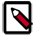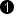## Operator SCALE

The operator performs a multiplication of the input with a parameterizable value. The multiplicand can be defined using parameter ScaleFactor. The range of ScaleFactor is defined by parameter ScaleFactorMaxBits if ScaleFactor is a dynamic parameter. Only positive values are allowed for the scale factor.

Less Resources for Static ParameterThe operator requires less FPGA resources if the parameter ScaleFactor is set to static. Moreover, users should consider using operator HWMULT together with CONST to save resources.

### I/O Properties

Property Value
Operator Type O
Input Link I, data input
Output Link O, data output

### Supported Link Format

Bit Width [1, 32] unsigned, [2, 32] signed autoArithmetic {unsigned, signed} as I
Parallelism any as I
Kernel Columns any as I
Kernel Rows any as I
Img Protocol {VALT_IMAGE2D, VALT_LINE1D, VALT_PIXEL0D} as I
Color Format VAF_GRAY as I
Color Flavor FL_NONE as I
Max. Img Width any as I
Max. Img Height any as I
 The bit width at the output is the input bit width + parameter ScaleFactorMaxBits if parameter ScaleFactor is set to dynamic. If the parameter is set to static, the output bit width is

### Parameters

ScaleFactorMaxBits
Type static parameter
Default 8
Range [1, 32]

Using this parameter, the range of parameter ScaleFactor is defined. If ScaleFactor is set to static, this parameter is disabled

ScaleFactor
Type static/dynamic read/write parameter
Default 1
Range [0, 2^ScaleFactorMaxBits - 1] if dynamic

This parameter defines the factor for the multiplication with the operator's input. The range of this parameter is [0, 1^ScaleFactorMaxBits - 1], if parameter ScaleFactor is dynamic. Otherwise, the range of this parameter is [1, 2^32 - 1].

### Examples of Use

The use of operator SCALE is shown in the following examples:

• ' Multiple DMA Channel Designs '

Threshold binarization

• 'Histogram Threshold'

Example - Histogram thresholding

• 'Blob 2D'

Examples - Shows the usage of operator Blob_Analysis_2D. The applet binarizes the input data and determines the blob analysis results. The results as well as the original image are output using two DMA channels.

• 'Blob2D ROI Selection'

Examples - The blob analysis operator is applied to an input camera image. The applet shows the usage of the blob data in the applet. In this case, the object with the maximum are is localized and the coordinates are used to cut out the object from the original image.

• 'Color Plane Separation Option 5 - Sequential Output with Advances Processing'

Example on separation of color planes. The RGB input is split into its component and sequentially output via one DMA channel. The splitting if performed by collecting same components in parallel words and reading with FrameBufferRandomRead.

• 'Kirsch Filter'

Examples - The Kirsch filter is a good edge detection filter for non directional edges.

• 'Roberts Cross Gradient'

Examples - Roberts Cross Gradient filter example.

• 'Sobel Gradient X'

Examples - A Sobel filter in x-direction only.

• 'Sobel Multi Gradient'

Examples - A Sobel filter in all 4 directions.

• 'Close'

Examples - Shows the implementation of a morphological close applied to binary images.

• 'Open'

Examples - Shows the implementation of a morphological open applied to binary images.

• 'Averaging 3x3'

Examples - A simple 3x3 box filter.

• 'Gaussian Filter 5x5'

Examples - A Gauss filter using a 5x5 kernel.

• 'Filter Sub Kernels'

Examples - Shows how to extract a sub kernel from a filter to obtain the original image data. This example performs a simple local adaptive binarization.

• 'High Boost Sharpening Filter'

Examples - A high boost Laplace filter for sharpening

• 'Laplace Filter 3x3'

Examples - A 3x3 Laplace filter.

• 'Downsampling 3x3'

Examples - Downsampling by factor 3x3 without the use of operator SampleDn.